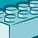Announcements
Sept. 27, 10AM ET Do more with Qlik, Insight Advisor – our intelligent AI-assistant in Qlik Sense: Register
cancel
Showing results for
Did you mean:Contributor II

## Sum total as dimension \$variable

Hello.
Where is the mistake I make?

\$vDoc is variable that contains dimension in my expression for documents no.

Sum(total <\$(vDoc)> Amount)

1 Solution

Accepted SolutionsContributor II
Author

Solution was to split my tables, then concatenate with multiple  where statement so I don't have unnecessary fetched results. Then I can use Sum function in bar chart with dimension shipment date.

5 RepliesCreator

Hi Veselin

I think you are doing some mistake in variable script, i am using the same script for sum calculation below is the example for your reference.

Let vCurrentYear = '{' & Chr(36) & '<Cal_Year={' & Chr(36) & '(=Max(Cal_Year))}>}';

SUM(Total \$(vCurrentYear)POLH_UW_CONTRIBUTION)

If still not work please share the script what you have written in backendContributor II
Author

Hi Nasir

set vDoc =

if ([Document Type] = '1' and BlankOrderNo='' and NoSeries <>'SR_CLAIM', [Document No_],

if ([Document Type]='4' and NoSeries <>'SR_CLAIM', [Document No_],null()));

This is working, because I'm using vDoc as dimension in my table.

And vDoc is showing me the correct Documents in my table.

But also I need to use this dimension in bar chart for properly calculation the amount.

So that's why I use this as expression but it's not working.Contributor II
Author

Solution was to split my tables, then concatenate with multiple  where statement so I don't have unnecessary fetched results. Then I can use Sum function in bar chart with dimension shipment date.Contributor

I would like to make a loop that will retrieve the data of the last 4 weeks via a sql request and store them in a qvd file per week.

Here is what a collegue did, but it doesn't work.

set jour_calcul = today();

let ann = year (\$(jour_calcul) - 45);

let ann_prec = \$(ann) - 1;

let mois = month (\$(jour_calcul) - 45);

// Chargt des fichiers

// -------------------

For j = 1 to 2

if j = 1 then

`` let sem_ref = \$(ann_prec) & '2'; let sem_enr = \$(ann) & '1'; let deb =\$(#mois); ``

else

`` let sem_ref = \$(ann) & '1'; let sem_enr = \$(ann) & '2'; if \$(#mois) < 7 then ``

let deb = 1;

else

`` let deb = \$(#mois) - 6; ``

end if

end if

For i = \$(#deb) to 6

set filename = SEGM_\$(sem_enr)_\$(i).qvd;

segm:

SQL request (just an example)

store segm into \$(filename) (qvd);

drop table segm;

next i

next jContributor II
Author